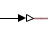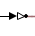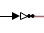Convert Simulink input signal into physical signal

•Libraries:
Simscape / Utilities

## Description

The Simulink-PS Converter block converts the input Simulink® signal into a physical signal. Use this block to connect Simulink sources or other Simulink blocks to the inputs of a Simscape™ physical network.

### Block Icon Display on the Model Canvas

To convey signal conversion while taking up minimal canvas space, the block icon changes dynamically based on whether it is connected to other blocks.

When Block Is...Block Icon

UnconnectedConnected to other blocksThe block icon also changes based on the value of the Input filtering order parameter, to indicate whether filtering is being applied to the input signal.

Input Filtering OrderUnconnected Block IconConnected Block Icon

`First-order filtering``Second-order filtering`### Unit Conversion and Checking

Simscape unit manager automatically handles unit propagation and checking within a physical network and performs the necessary unit conversion operations.

The physical signal at the output port of the Simulink-PS Converter block serves as an input signal for the Simscape physical network that the block is connected to. The physical signal unit must be commensurate with the unit expected by the input port of the destination block, that is, the input port connected to the output port of the Simulink-PS Converter block.

Simulink signal units do not propagate into physical networks. The Input signal unit parameter lets you specify a physical unit for the input signal value, so that the Simscape unit manager can perform the necessary unit conversions and scale the output physical signal accordingly.

Note

If you also specify a physical unit as an attribute of the Simulink signal connected to the input port of the block, the software checks that the two units match. For more information, see Working with Simulink Units.

In other words, the Input signal unit parameter does not determine the units of the output physical signal, it only provides a scaling value. The output physical signal unit is inferred from the destination block. The default destination block units are meter-kilogram-second or MKS (SI). If you leave the Simulink-PS Converter block unitless, with the Input signal unit parameter set to `1`, then the block does not apply scaling to the input signal. If you specify different units, commensurate with the expected default units of the destination block input, then the unit manager attaches these units to the input Simulink signal value and performs the necessary unit conversion when providing the signal to the destination block.

In the diagram below, the Ideal Torque Source block expects a torque signal, in N*m, on its S port. The Constant source block provides the value for this input signal. If you left the Simulink-PS Converter block unitless, the Ideal Torque Source block would generate torque of 1000 N*m. The parameters of other blocks in this example are chosen so that the output value of the Ideal Torque Sensor block is equal to the torque generated by the Ideal Torque Source block, and therefore the Display block would show the value of 1000. If you change the Input signal unit parameter value in the Simulink-PS Converter block to `N*cm`, the unit manager performs the conversion and the Ideal Torque Source block generates torque of 10 N*m; the torque value in the Display block changes to 10, as shown in the diagram.When the input signal is related to thermodynamic variables and contains units of temperature, you must decide whether affine conversion needs to be applied. For more information, see When to Apply Affine Conversion. Usually, if the input signal represents a relative temperature, that is, a change in temperature, you need to apply linear conversion, ΔTnew = L * ΔTold (the default method). However, if the input signal represents an absolute temperature, you need to apply affine conversion, Tnew = L * Told + O.

For example, in the Simulink-PS Converter block shown in the following diagram, if you type `degC` in the Input signal unit field and select the Apply affine conversion check box, the temperature generated by the Ideal Temperature Source block is equal to 293.15 K. However, if you leave the Apply affine conversion check box clear, the output of the Ideal Temperature Source block is 20 K.Note

Untyped inputs do not support affine units. If you specify affine units in a Simulink-PS Converter block and then connect it directly to an untyped input port, the signal value is converted to the corresponding fundamental unit and further mathematical operations are performed with that value.

### Input Handling

When simulating a model, you may need to provide time derivatives of some of the input signals, especially if you use an explicit solver. One way of providing the necessary input derivatives is by filtering the input through a low-pass filter. Input filtering makes the input signal smoother and generally improves model performance. The additional benefit is that the Simscape engine computes the time derivatives of the filtered input. The first-order filter provides one derivative, while the second-order filter provides the first and second derivatives. If you use input filtering, it is very important to select the appropriate value for the filter time constant.

The filter time constant controls the filtering of the input signal. The filtered input follows the true input but is smoothed, with a lag on the order of the time constant that you choose. Set the time constant to a value no larger than the smallest time interval in the system that interests you. If you choose a very small time constant, the filtered input signal is closer to the true input signal. However, this filtered input signal increases the stiffness of the system and slows the simulation.

Instead of using input filtering, you can provide time derivatives for the input signal directly, as additional Simulink signals. If the provided derivatives are inconsistent with the input signal, then some of the quantities may be incorrect during simulation.

For piecewise-constant signals, you can also explicitly set the input derivatives to zero. Use this option for signals that are truly piecewise-constant, such as step. If you have a continuous input signal sampled with a discrete sample time, setting input derivatives to zero can produce incorrect simulation results. Use one of the other two options: either filter the input or provide time derivatives as separate signals.

## Ports

### Input

expand all

Input Simulink signal that the block converts into the output physical signal.

Data Types: `double`

Simulink signal that provides the first derivative of the input signal at Port_1.

#### Dependencies

To enable this port, set the Provided signals parameter to `Input and first derivative` or ```Input and first two derivatives```.

Data Types: `double`

Simulink signal that provides the second derivative of the input signal at Port_1.

#### Dependencies

To enable this port, set the Provided signals parameter to `Input and first two derivatives`.

Data Types: `double`

### Output

expand all

Output physical signal. The signal size matches the size of the input signal at Port_1. The signal unit is determined by the destination block. The Input signal unit parameter and Apply affine conversion check box let you apply scaling and linear offset to the input signal value to calculate the correct value of the output signal.

## Parameters

expand all

### Units

Units to be assigned to the input Simulink signal, to let the unit manager perform the necessary unit conversion and scale the signal value accordingly. These units must be commensurate with the expected default units of the destination block input. You can select a unit from the drop-down list, or type the desired unit name, such as `rpm`, or a valid expression, such as `mm/s`. For more information and a list of unit abbreviations, see How to Specify Units in Block Dialogs and Unit Definitions. The default value is `1`, which means that no scaling is applied. The physical signal at the block output matches the value of the input Simulink signal at Port_1, in the units expected by the destination block inside the physical network.

This check box is applicable only for units that can be converted either with or without an affine offset, such as `degC` or `degF`. Select this check box if the input signal represents absolute temperature in degrees Celsius or degrees Fahrenheit. For more information, see Thermal Unit Conversions.

### Input Handling

This parameter lets you decide whether to provide time derivatives of the input signal through additional input ports or by filtering:

• `Provide signals` — Select whether you want to provide just the input signal, or also provide time derivatives of the input signal as additional input signals, by using the Provided signals parameter. The default input handling options are `Provide signals` and ```Input only```. If you use an explicit solver, it is recommended that you provide input derivatives by selecting one of the other options. For more information, see Filtering Input Signals and Providing Time Derivatives.

• `Filter input, derivatives calculated` — Filter the input through a low-pass filter, which also provides input derivatives. In this case, the input signal is modified (through filtering) before being converted to a physical signal. The first-order filter provides one derivative, while the second-order filter provides the first and second derivatives. If you use this option, set the appropriate Input filtering time constant parameter value.

• `Zero derivatives (piecewise constant)` — If your input signal is piecewise constant (such as step), this option lets you explicitly set the input derivatives to zero.

This parameter lets you provide time derivatives of the input signal as additional input signals:

• `Input only` — Provide just the input signal. This is the default. If you select this option, the block has one Simulink input port and one physical signal output port.

• `Input and first derivative` — If you select this option, an additional Simulink input port appears on the Simulink-PS Converter block, to let you connect the signal providing input derivative.

• `Input and first two derivatives` — If you select this option, two additional Simulink input ports appear on the Simulink-PS Converter block, to let you connect the signals providing input derivatives.

#### Dependencies

To enable this parameter, set Filtering and derivatives to `Provide signals`.

This parameter lets you specify the number of time derivatives of the input signal provided by filtering:

• `First-order filtering` — Provides only the first derivative.

• `Second-order filtering` — Provides the first and second derivatives.

#### Dependencies

To enable this parameter, set Filtering and derivatives to `Filter input, derivatives calculated`.

Specify the filter time constant, in seconds, which controls the filtering of the input signal. The filtered input follows the true input but is smoothed, with a lag on the order of the time constant. When you select a value for this parameter, consider the system dynamics and set the time constant to a value no larger than the smallest time interval of interest during simulation. The trade-off in choosing a very small time constant is that the filtered input signal will be closer to the true input signal, at the cost of increasing the stiffness of the system and slowing down the simulation.

#### Dependencies

To enable this parameter, set Filtering and derivatives to `Filter input, derivatives calculated`.

## Version History

Introduced in R2007a